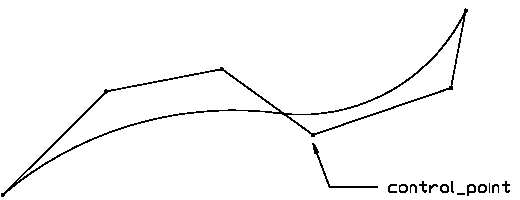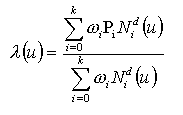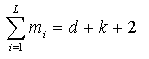# IFC 4.3.x development

## 8.9.3.5 IfcBSplineCurve

### Change log

#### IFC4

 additions where_rules SameDim deletions where_rules WR41 modifications attributes ClosedCurve definition Changed from logical to IfcLogical modifications attributes Degree definition Changed from integer to IfcInteger modifications attributes SelfIntersect definition Changed from logical to IfcLogical

### 8.9.3.5.1 Semantic definitions at the entity

The IfcBSplineCurve is a spline curve parameterized by spline functions.

Figure 1 illustrates a B-spline curve.

NOTE  Figure quoted from ISO 10303-42.NOTE  Definition according to ISO/CD 10303-42:1992
A B-spline curve is a piecewise parametric polynomial or rational curve described in terms of control points and basis functions. The B-spline curve has been selected as the most stable format to represent all types of polynomial or rational parametric curves. With appropriate attribute values it is capable of representing single span or spline curves of explicit polynomial, rational, Bezier or B-spline type.

Interpretation of the data is as follows:

1. All weights shall be positive and the curve is given byk+1 = number of control points Pi = control points wi = weights d = degree

The knot array is an array of (k+d+2) real numbers [u-d ... uk+1], such that for all indices j in [-d,k], uj <= uj+1. This array is obtained from the knot data list by repeating each multiple knot according to the multiplicity. N di, the ith normalized B-spline basis function of degree d, is defined on the subset [ui-d, ... , ui+1] of this array.

2. Let L denote the number of distinct values among the d+k+2 knots in the knot array; L will be referred to as the 'upper index on knots'. Let mj denote the multiplicity (number of repetitions) of the jth distinct knot. ThenAll knot multiplicities except the first and the last shall be in the range 1 ... degree; the first and last may have a maximum value of degree + 1. In evaluating the basis functions, a knot u of e.g. multiplicity 3 is interpreted as a string u, u, u, in the knot array. The B-spline curve has 3 special subtypes (Note: only 1, Bezier curve, included in this IFC release) where the knots and knot multiplicities are derived to provide simple default capabilities.

3. Logical flag is provided to indicate whether the curve self intersects or not.
4. NOTE  Entity adapted from b_spline_curve defined in ISO10303-42.

HISTORY  New entity in IFC2x2.

### 8.9.3.5.1 Attributes

# Attribute Type Description
IfcRepresentationItem
LayerAssignment SET [0:1] OF IfcPresentationLayerAssignment FOR AssignedItemsAssignment of the representation item to a single or multiple layer(s). The LayerAssignments can override a LayerAssignments of the IfcRepresentation it is used within the list of Items.

> IFC2x3 CHANGE  The inverse attribute LayerAssignments has been added.

> IFC4 CHANGE  The inverse attribute LayerAssignment has been restricted to max 1. Upward compatibility for file based exchange is guaranteed.
StyledByItem SET [0:1] OF IfcStyledItem FOR Item Reference to the IfcStyledItem that provides presentation information to the representation, e.g. a curve style, including colour and thickness to a geometric curve.

> IFC2x3 CHANGE  The inverse attribute StyledByItem has been added.
IfcGeometricRepresentationItem
IfcCurve
Dim INVALID The space dimensionality of this abstract class, defined differently for all subtypes, i.e. for IfcLine, IfcConic and IfcBoundedCurve.
IfcBoundedCurve
IfcBSplineCurve
1 Degree IfcInteger The algebraic degree of the basis functions.
2 ControlPointsList LIST [2:?] OF IfcCartesianPoint The list of control points for the curve.
3 CurveForm IfcBSplineCurveForm Used to identify particular types of curve; it is for information only.
4 ClosedCurve IfcLogical Indication of whether the curve is closed; it is for information only.
5 SelfIntersect IfcLogical Indication whether the curve self-intersects or not; it is for information only.
UpperIndexOnControlPointsINVALID The upper index on the array of control points; the lower index is 0.

This value is derived from the control points list.
ControlPoints INVALID The array of control points used to define the geometry of the curve. This is derived from the list of control points.

### 8.9.3.5.3 Formal representations

```ENTITY IfcBSplineCurve
ABSTRACT SUPERTYPE OF (ONEOF
(IfcBSplineCurveWithKnots))
SUBTYPE OF (IfcBoundedCurve);
Degree : IfcInteger;
ControlPointsList : LIST [2:?] OF IfcCartesianPoint;
CurveForm : IfcBSplineCurveForm;
ClosedCurve : IfcLogical;
SelfIntersect : IfcLogical;
DERIVE
UpperIndexOnControlPoints : IfcInteger := (SIZEOF(ControlPointsList) - 1);
ControlPoints : ARRAY [0:UpperIndexOnControlPoints] OF IfcCartesianPoint := IfcListToArray(ControlPointsList,0,UpperIndexOnControlPoints);
WHERE
SameDim : SIZEOF(QUERY(Temp <* ControlPointsList |
Temp.Dim <> ControlPointsList.Dim))
= 0;
END_ENTITY;```